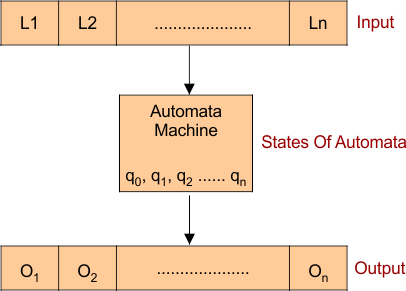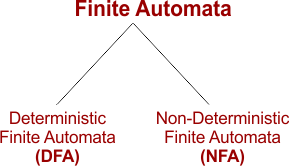Select Page

# Introduction To Finite Automata

Finite Automata (FA) is a machine which accepts all the regular languages. It is used to recognize the patterns. It is also called finite state machine.

## Working Of Finite Automata

• The finite automata machine contains five tuple or elements.
• It has some finite states and rules for moving from one state to another but it depends upon the given input symbol.

Basic diagram is given belowA Finite Automata can be defined through its 5 tuples { Q, Σ, q, F, δ }. Where

• Q : Finite set of states.
• Σ : set of Input Symbols.
• q : Initial state.
• F : set of Final States.
• δ : Transition Function.

## Types Of Finite Automata

FA is characterized into two types:### 1) Deterministic Finite Automata (DFA)

• In a DFA, for a particular input symbol, the machine goes to one state only.
• In DFA null (ε) move is not allowed. It means state cannot change without a input symbol.

DFA contains 5 tuples {Q, Σ, q, F, δ}.

Q : set of all states.

Σ : set of input symbols.

q : Initial state.

F : set of final state.

δ : Transition Function, δ : Q X Σ –> Q.

### 2) Nondeterministic Finite Automata(NFA)

• NFA is similar to DFA except the Null (or ε) move. In NFA Null move is allowed. It means state can change without a input symbol.
• NFA also has similar 5 tuples {Q, Σ, q, F, δ} like DFA except transition function. It NFA Transition Function is given below

δ:  Q X (Σ U ε ) –> 2 ^ Q.

## Some important points about DFA and NFA

• Every DFA is NFA, but NFA is not DFA.
• In NFA and DFA, there can be multiple final states.
• DFA is used in Lexical Analysis in Compiler.
• NFA mostly used as theoretical concept.
Help Other’s By Sharing…